﻿ LOESS用于GNSS垂向坐标时间序列噪声识别与提取文章快速检索 高级检索
 大地测量与地球动力学2021, Vol. 41Issue (10): 1024-1029  DOI: 10.14075/j.jgg.2021.10.007引用本文CHEN Xiang, YANG Zhiqiang, YANG Bing, et al. Noise Recognition and Extraction of GNSS Vertical Coordinate Time Series Based on LOESS[J]. Journal of Geodesy and Geodynamics, 2021, 41(10): 1024-1029.Foundation support

Natural Science Foundation of Shaanxi Province, No.2019JQ-668; Key Research and Development Program of Shaanxi Province, No.2018SF-381.

Corresponding author

YANG Zhiqiang, PhD, professor, PhD supervisor, majors in crustal deformation monitoring and geodynamic, E-mail: yang_gps@chd.edu.cn.

第一作者简介

CHEN Xiang, postgraduate, majors in GNSS data processing, E-mail: 1689482074@qq.com.

文章历史

LOESS用于GNSS垂向坐标时间序列噪声识别与提取

1. 长安大学地质工程与测绘学院，西安市雁塔路126号，710054

1 LOESS原理及降噪方法 1.1 LOESS方法基本原理

LOESS是一种基于局部回归分析的非参数降噪方法，与线性回归、多项式回归等方法相比，该方法未对预测点进行限制，而是直接基于数据进行分析。该方法需要预先设置一个窗口，根据窗口内的点计算拟合点的拟合值，具体过程如下：

1) 对坐标时间序列中第1个点(x1, y1)进行拟合，此时窗口位于坐标时间序列的最左端，计算对应窗口内所有点相对于(x1, y1)的权重，通过加权最小二乘进行二次多项式拟合，得到回归曲线并计算得到拟合后的点(x1, $\hat y$1)。

2) 设(xk, yk)为窗口在最左端时的中心点，重复步骤1)，对(x1, y1)到(xk, yk)进行拟合，此时窗口位置固定，但从点(xk+1, yk+1)开始需要以拟合点为中心移动窗口位置，并逐个计算各点的拟合值，参与拟合值计算的仍为窗口内所有点。

3) 当窗口移动到坐标时间序列的最右端时，与最左端的情况类似，此时窗口中心点(xmk+1, ymk+1)到右端点(xm, ym)(m为坐标时间序列的总长度)均采用同一窗口内的所有点进行拟合。

4) 通过上述步骤对坐标时间序列中所有点进行拟合，将拟合后的点连接成完整的LOESS回归曲线，该曲线即为降噪后的坐标时间序列。

 $W\left( u \right) = \left\{ \begin{array}{l} {(1 - {u^3})^3}, {\rm{ }}0 \le u < 1\\ 0, u \ge 1 \end{array} \right.$ (1)

 ${\upsilon _i}\left( x \right) = W\left( {\frac{{|{x_i} - x|}}{{\Delta \left( x \right)}}} \right)$ (2)

 $J\left( \mathit{\boldsymbol{\theta }} \right) = \sum\limits_i {{\upsilon _i}\left( x \right){{({y_i} - {\mathit{\boldsymbol{\theta }}^{\rm{T}}}{x_i})}^2}}$ (3)

1.2 技术路线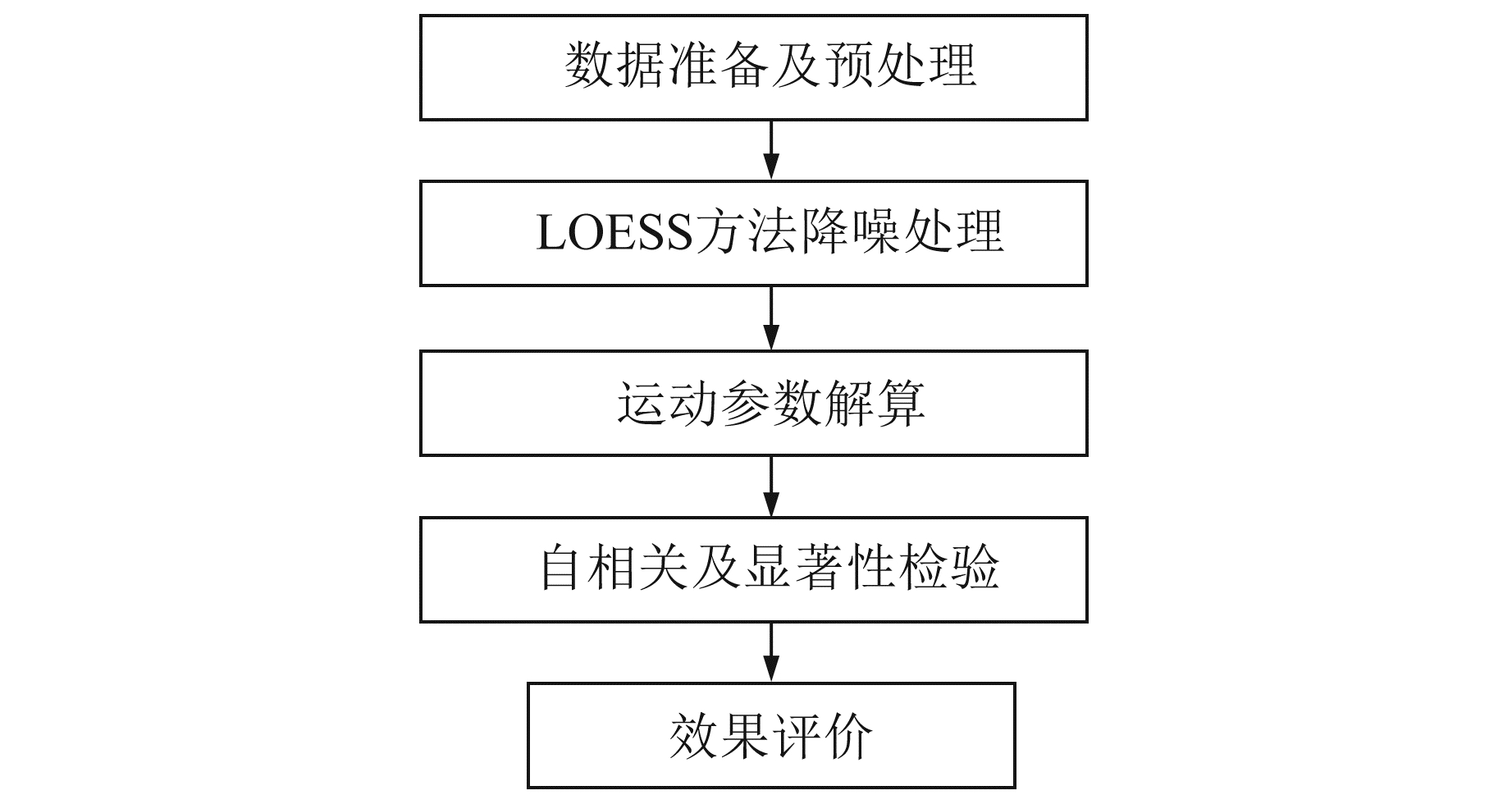图 1 技术路线 Fig. 1 Technical road
2 数据 2.1 数据来源

2.2 数据预处理

3 算例及结果分析 3.1 算例分析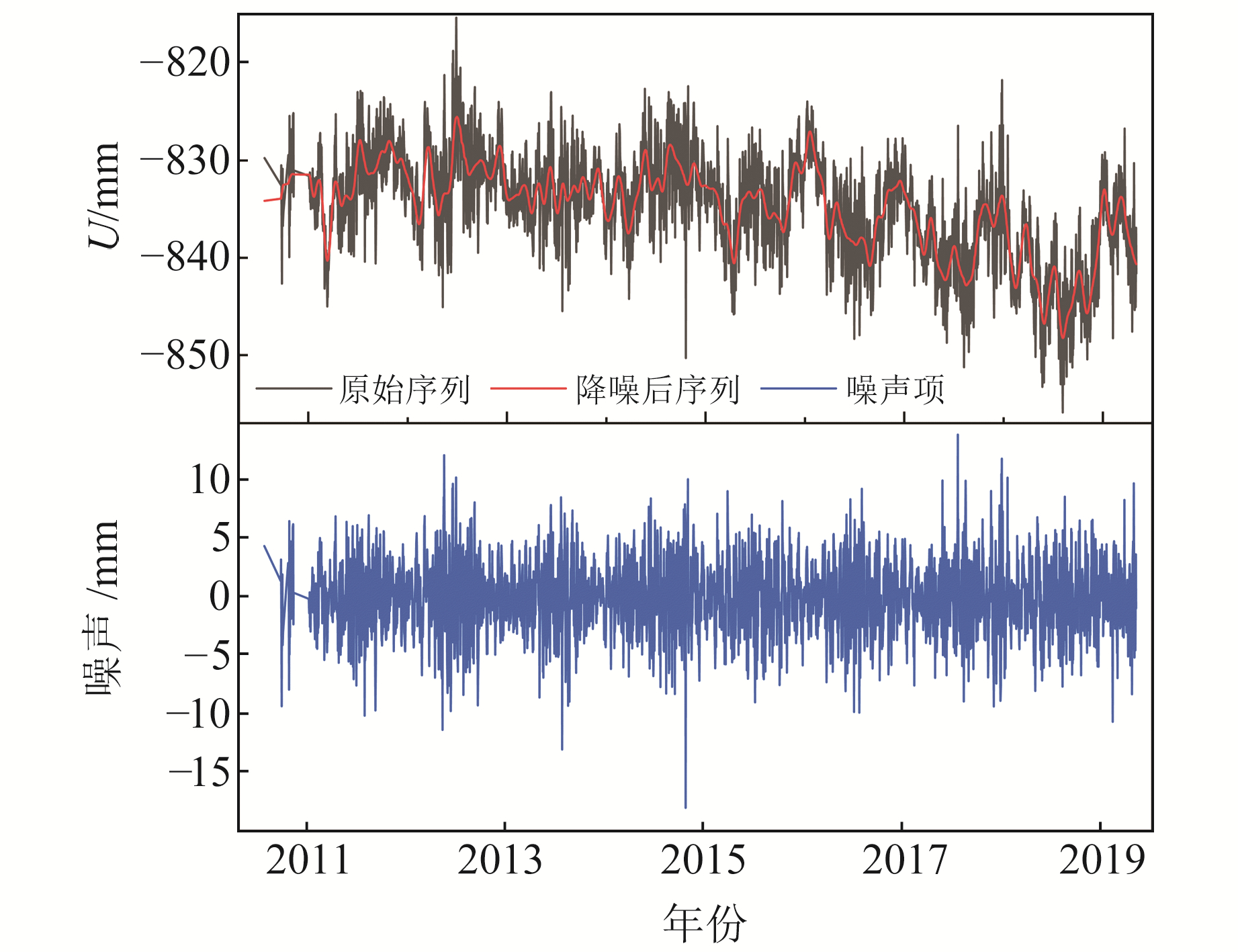图 2 XJHT站垂向GPS原始坐标时间序列、降噪后时间序列和噪声项 Fig. 2 Vertical GPS original coordinate time series, time series after noise reduction and noise items of XJHT station表 1 XJHT站降噪前后评价指标 Tab. 1 Evaluation index of XJHT station before and after noise reduction
3.2 自相关性检验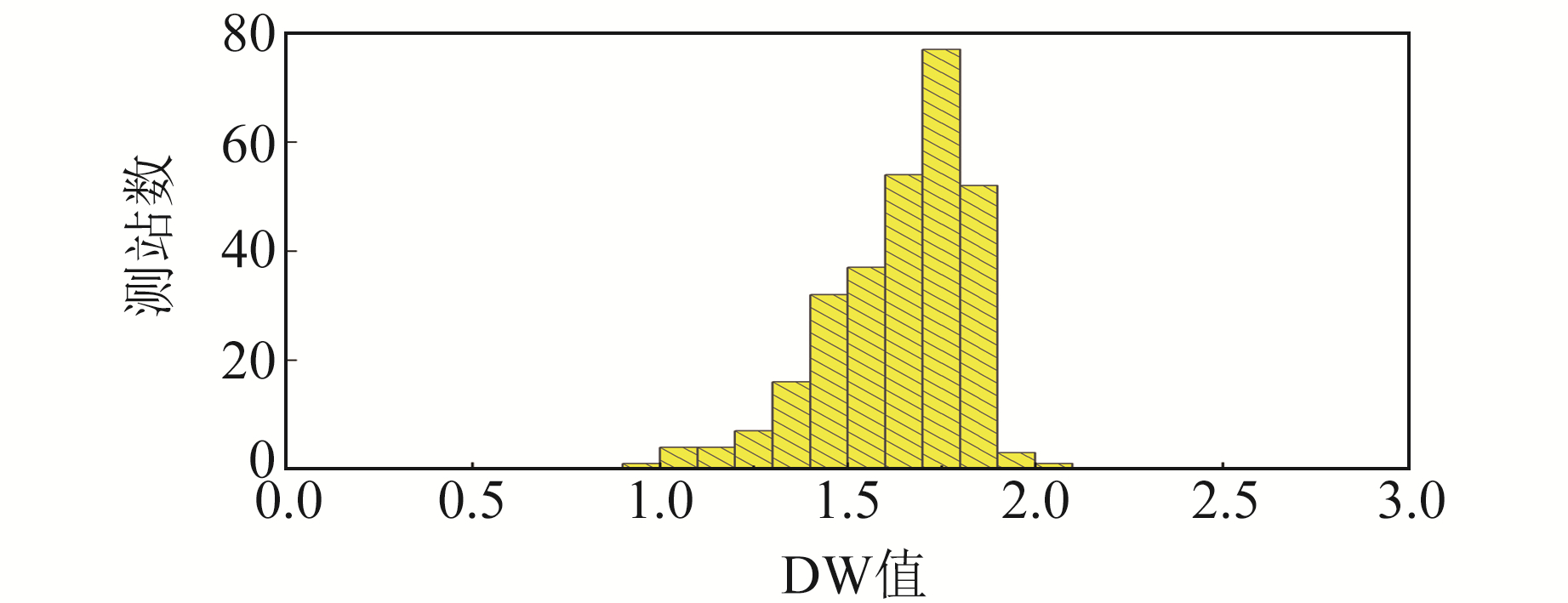图 3 DW检验结果 Fig. 3 DW test results
3.3 评价指标

3.3.1 信噪比

SNR计算公式可表示为：

 $\mathrm{SNR}=10 \log \left\{\frac{\sum\limits_{n=0}^{N-1} S_{n}^{2}}{\sum\limits_{n=0}^{N-1}\left(S_{n}-\hat{S}_{n}\right)^{2}}\right\}$ (4)

3.3.2 GNSS测站运动模型

 \begin{aligned} x(t)=& \sum\limits_{i=0}^{n_{P}} p_{i}\left(t-t_{R}\right)^{i}+\sum\limits_{j=1}^{n_{J}} b_{j} H\left(t-t_{j}\right)+\\ & \sum\limits_{k=1}^{n_{F}} s_{k} \sin \left(\omega_{k} t\right)+c_{k} \cos \left(\omega_{k} t\right)+\\ & \sum\limits_{m=1}^{n_{M}} a_{k} \log \left(1+\left(t-t_{m}\right) / T_{m}\right) \end{aligned} (5)

3.3.3 指标改正率

 ${\rm{CR}} = \frac{{{I_{{\rm{before}}}} - {I_{{\rm{after}}}}}}{{{I_{{\rm{before}}}}}} \times 100$ (6)

3.4 降噪效果评价

3.4.1 SNR与STD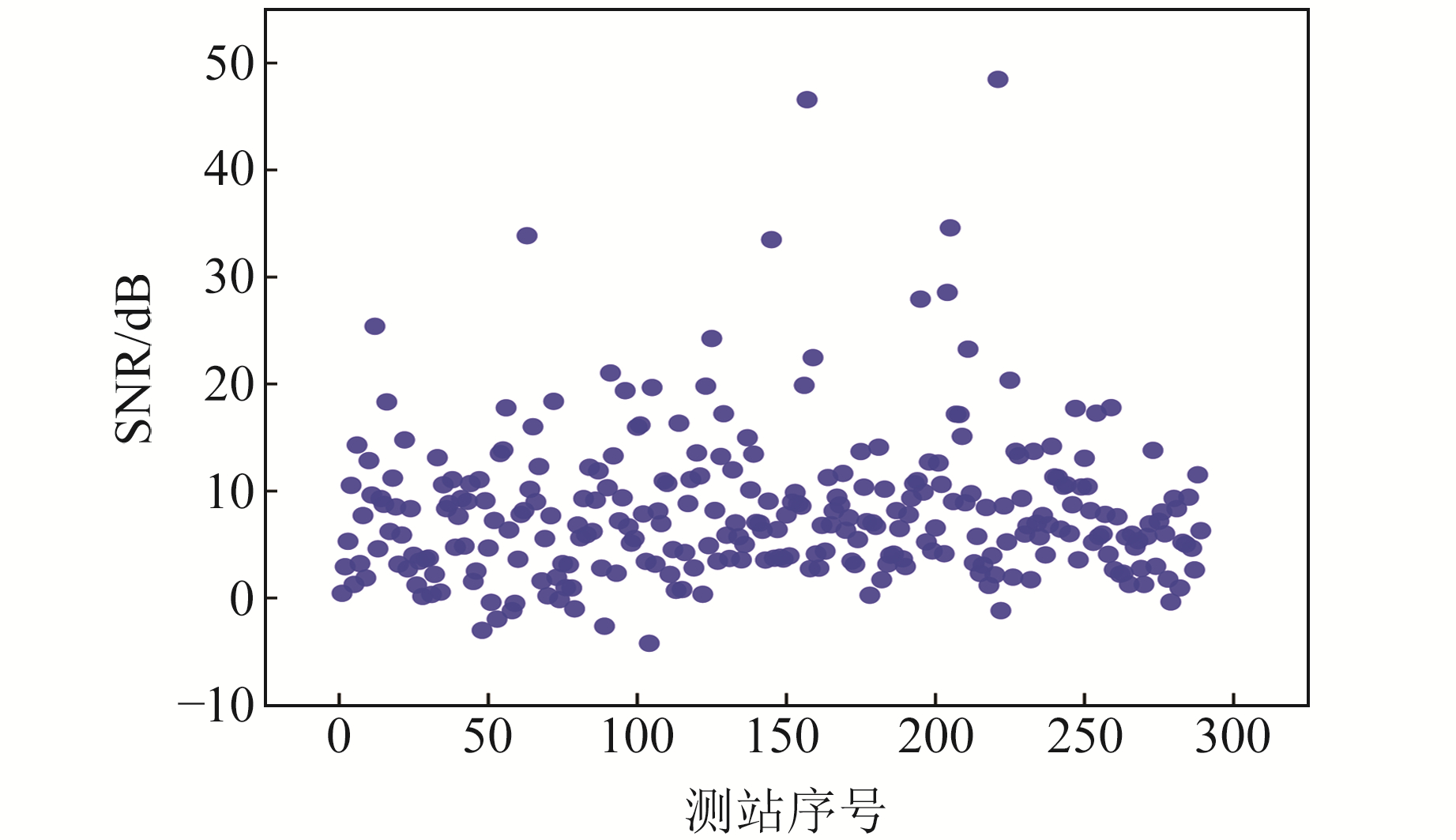图 4 各测站坐标时间序列降噪后的信噪比 Fig. 4 Signal-to-noise ratio after noise reduction in coordinate time series of each station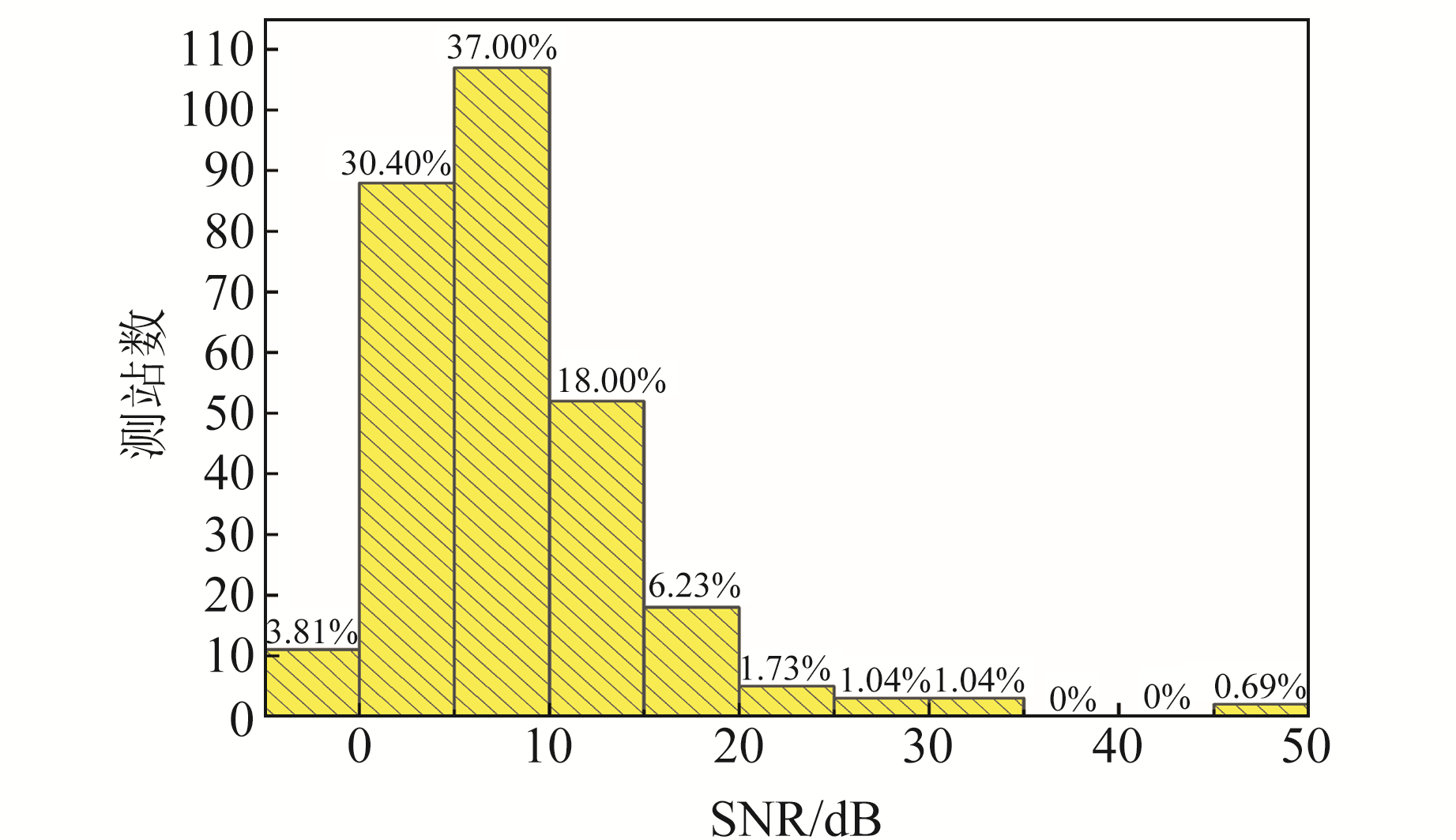图 5 降噪后信噪比的测站分布 Fig. 5 Distribution of stations for signal-to-noise ratio after noise reduction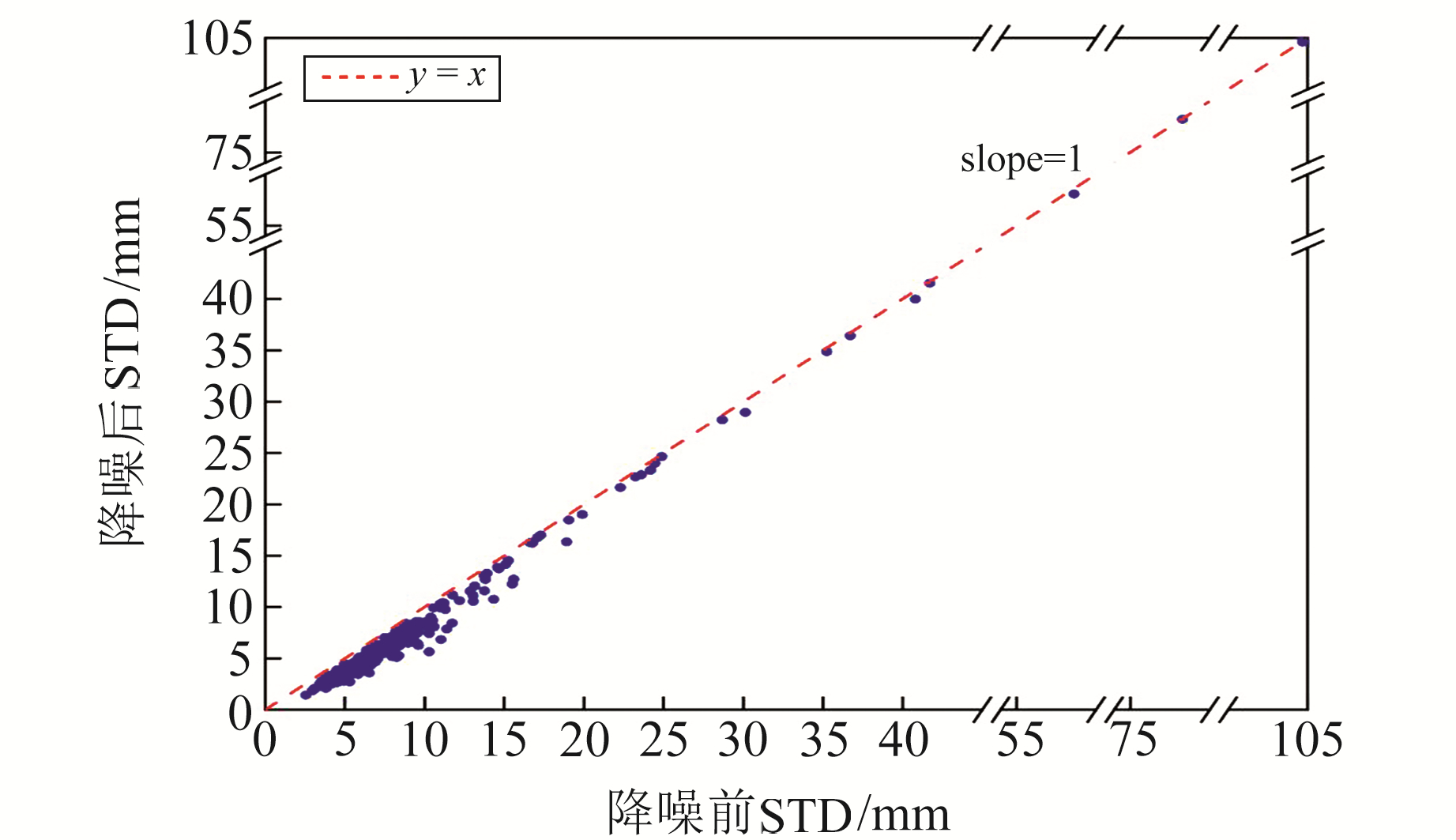图 6 降噪前后各测站序列的标准差 Fig. 6 The standard deviation of each station series before and after noise reduction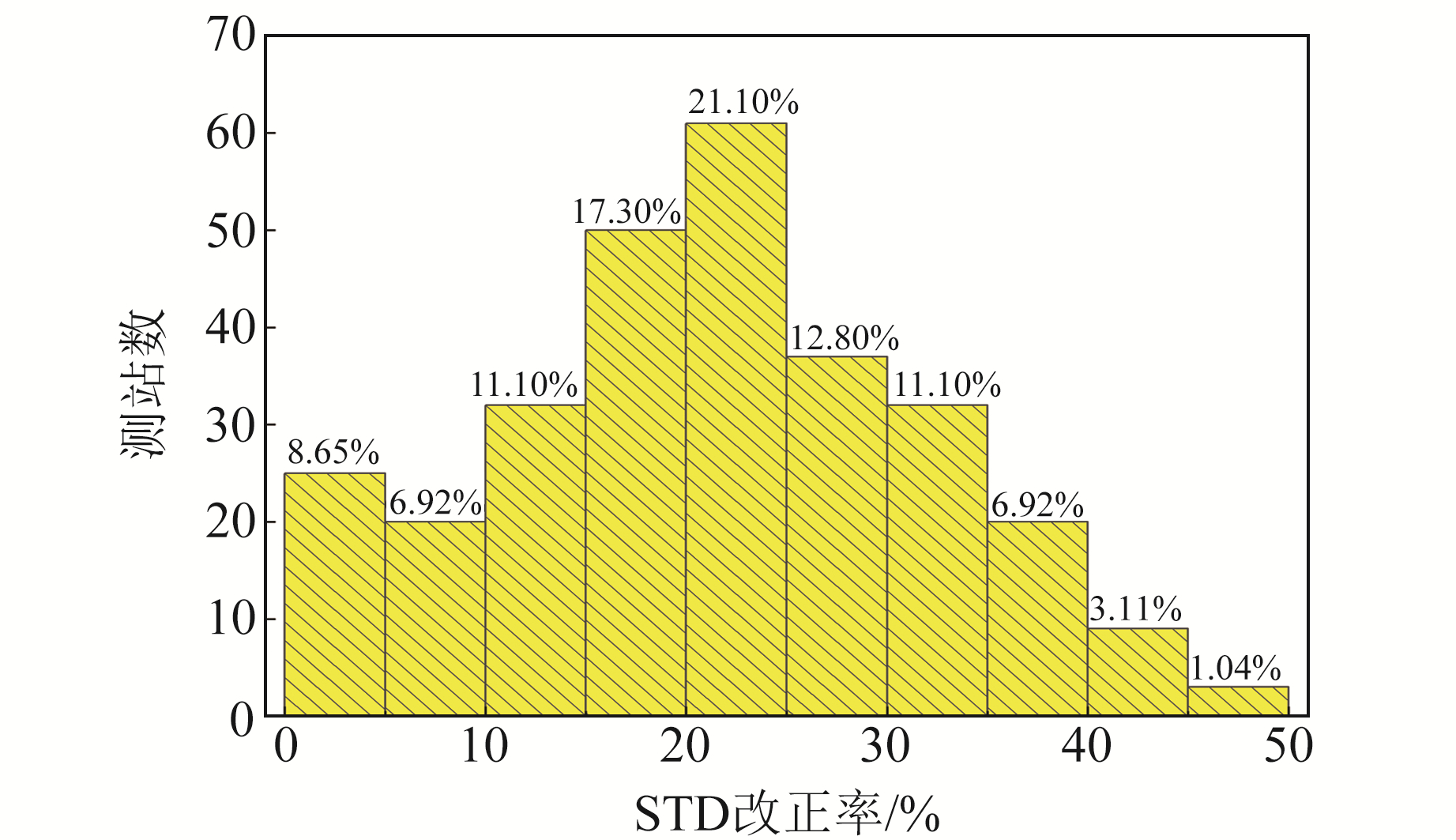图 7 标准差改正率的测站分布 Fig. 7 Distribution of stations for correction rate of standard deviation
3.4.2 运动模型参数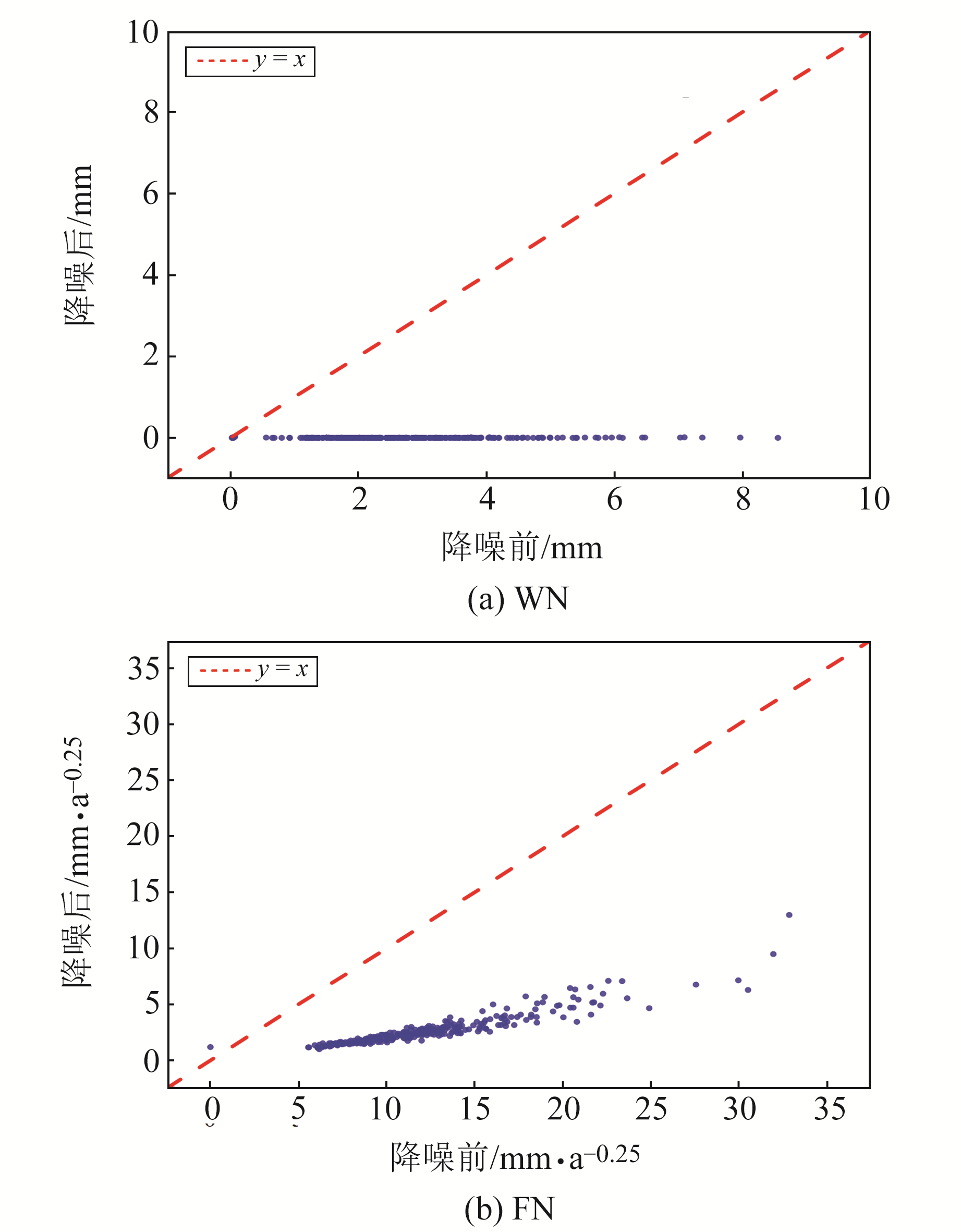图 8 降噪前后各测站坐标时间序列的白噪声和闪烁噪声 Fig. 8 White noise and flicker noise of coordinate time series of each station before and after noise reduction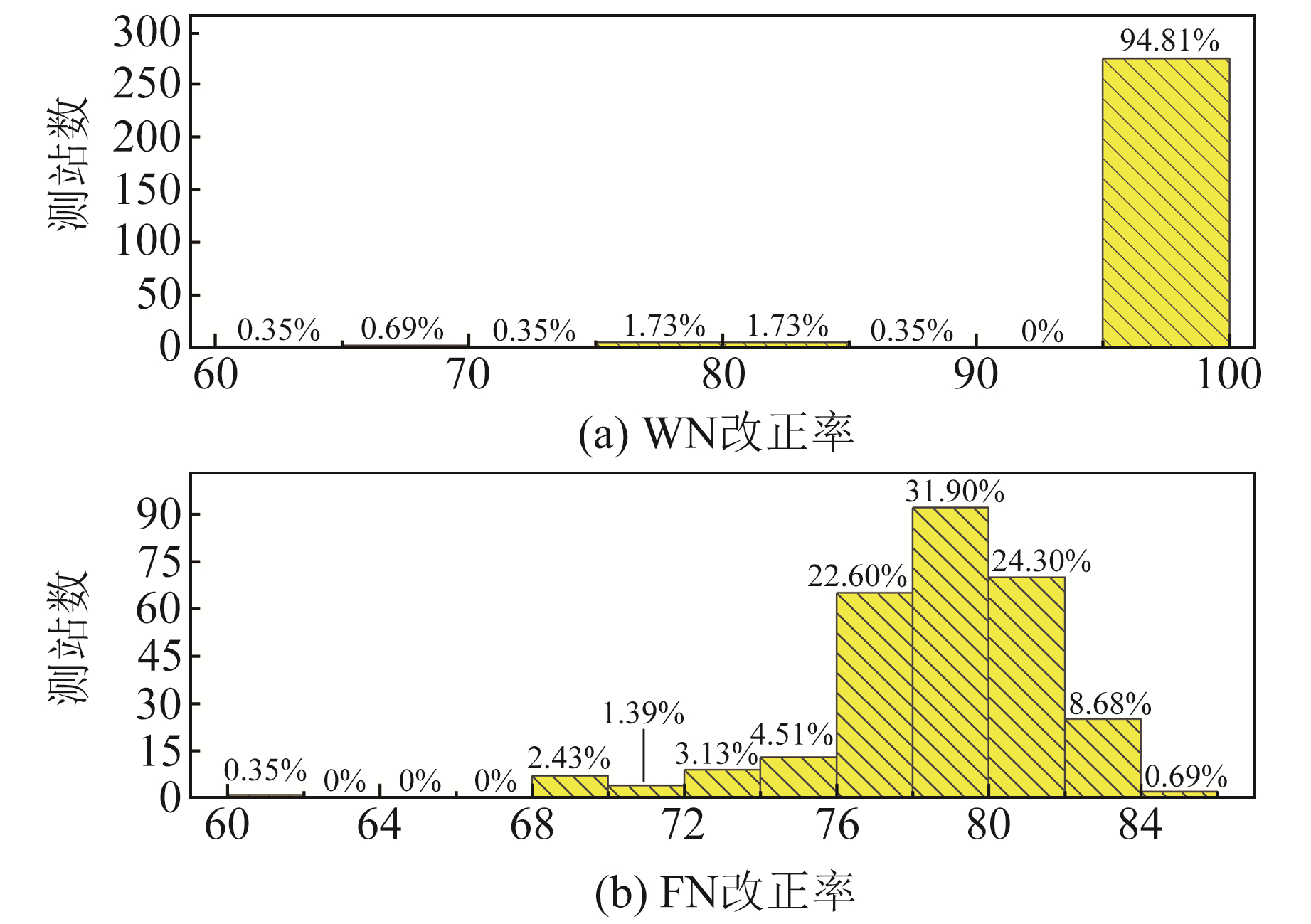图 9 白噪声和闪烁噪声改正率的测站分布 Fig. 9 Distribution of stations for correction rate of white noise and flicker noise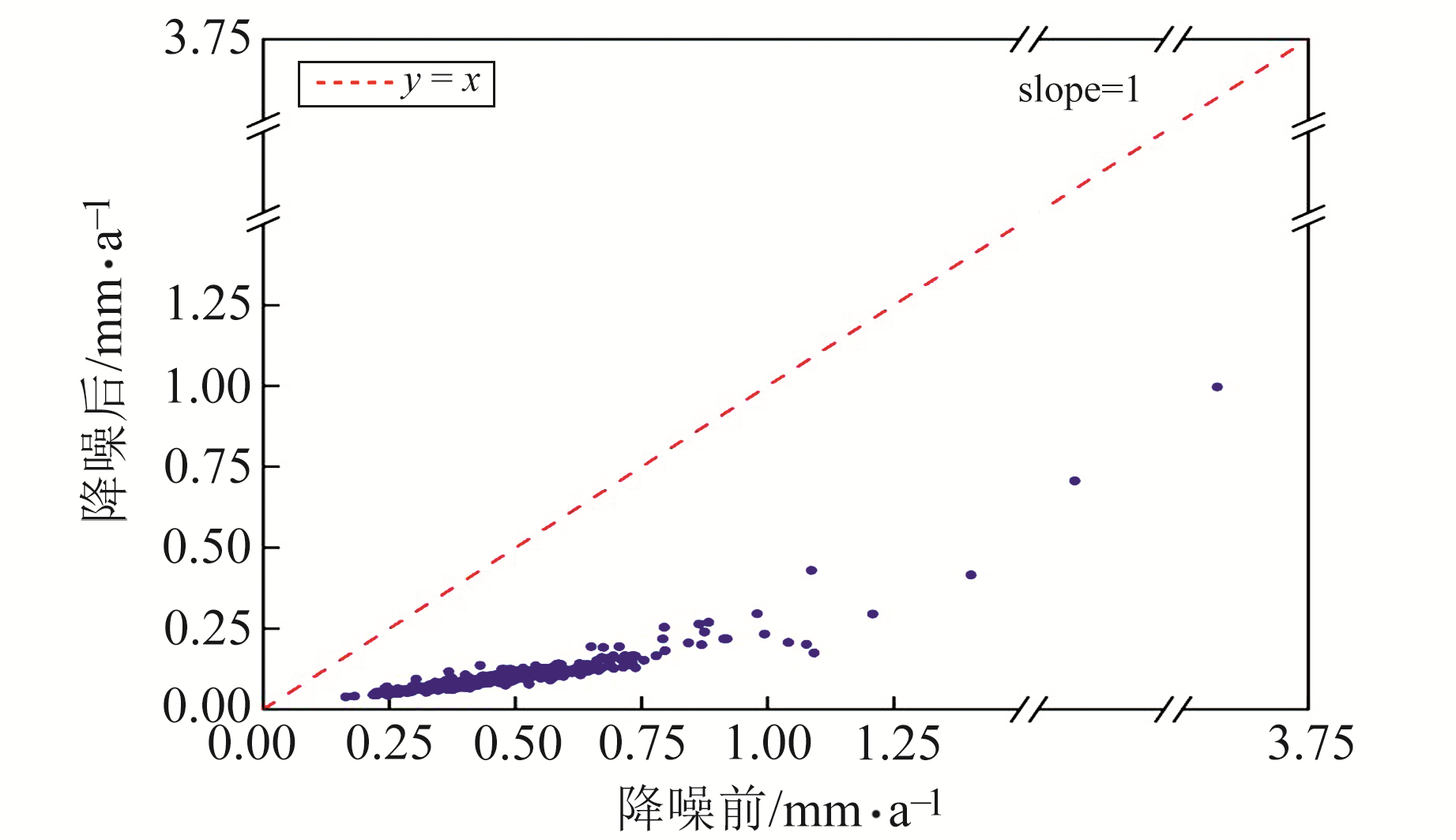图 10 降噪前后各测站坐标时间序列的速度不确定度 Fig. 10 The speed uncertainty of coordinate time series of each station before and after noise reduction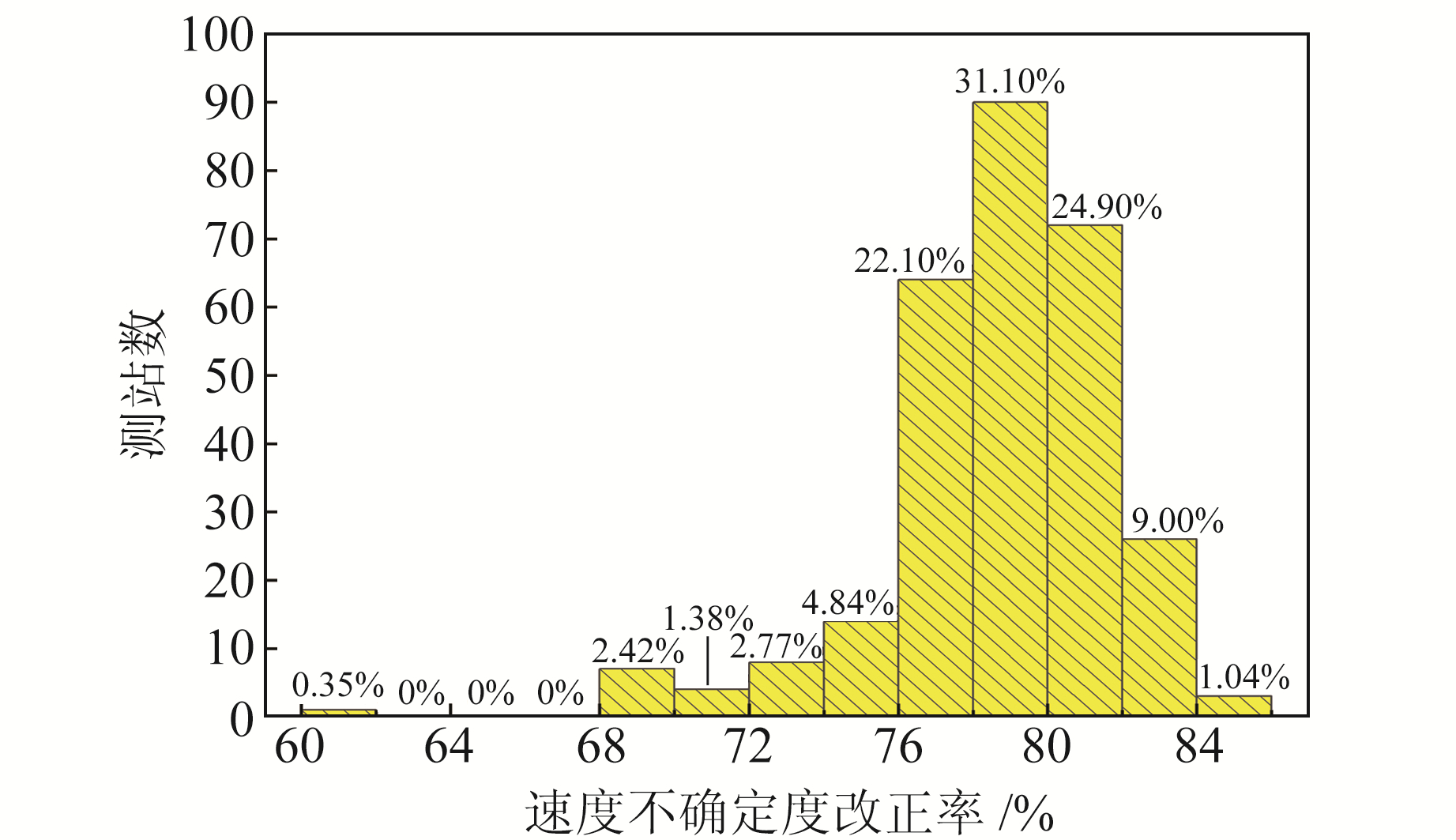图 11 速度不确定度改正率的测站分布 Fig. 11 Distribution of stations for correction rate of speed uncertainty
4 结语

  陈俊勇, 张鹏, 武军郦, 等. 关于在中国构建全球导航卫星国家级连续运行站系统的思考[J]. 测绘学报, 2007, 36(4): 366-369 (Chen Junyong, Zhang Peng, Wu Junli, et al. On Chinese National Continuous Operating Reference Station System of GNSS[J]. Acta Geodaetica et Cartographica Sinica, 2007, 36(4): 366-369 DOI:10.3321/j.issn:1001-1595.2007.04.002) (0)  甘卫军, 李强, 张锐, 等. 中国大陆构造环境监测网络的建设与应用[J]. 工程研究——跨学科视野中的工程, 2012, 4(4): 324-331 (Gan Weijun, Li Qiang, Zhang Rui, et al. Construction and Application of Tectonic and Environmental Observation Network of Mainland China[J]. Journal of Engineering Studies, 2012, 4(4): 324-331) (0)  姚宜斌, 张顺, 孔建. GNSS空间环境学研究进展和展望[J]. 测绘学报, 2017, 46(10): 1 408-1 420 (Yao Yibin, Zhang Shun, Kong Jian. Research Progress and Prospect of GNSS Space Environment Science[J]. Acta Geodaetica et Cartographica Sinica, 2017, 46(10): 1 408-1 420) (0)  李昭, 姜卫平, 刘鸿飞, 等. 中国区域IGS基准站坐标时间序列噪声模型建立与分析[J]. 测绘学报, 2012, 41(4): 496-503 (Li Zhao, Jiang Weiping, Liu Hongfei, et al. Noise Model Establishment and Analysis of IGS Reference Station Coordinate Time Series Inside China[J]. Acta Geodaetica et Cartographica Sinica, 2012, 41(4): 496-503) (0)  Wu H, Li K, Shi W Z, et al. A Wavelet-Based Hybrid Approach to Remove the Flicker Noise and the White Noise from GPS Coordinate Time Series[J]. GPS Solutions, 2015, 19(4): 511-523 DOI:10.1007/s10291-014-0412-6 (0)  鲁铁定, 钱文龙, 贺小星, 等. 一种确定分界IMF分量的改进EMD方法[J]. 大地测量与地球动力学, 2020, 40(7): 720-725 (Lu Tieding, Qian Wenlong, He Xiaoxing, et al. An Improved EMD Method for Determining Boundary IMF[J]. Journal of Geodesy and Geodynamics, 2020, 40(7): 720-725) (0)  Cleveland R B, Cleveland W S, McRae J E, et al. STL: A Seasonal-Trend Decomposition Procedure Based on Loess[J]. Journal of Official Statistics, 1990, 6(1): 3-73 (0)  Isotta F, Martius O, Sprenger M, et al. Long-Term Trends of Synoptic-Scale Breaking Rossby Waves in the Northern Hemisphere between 1958 and 2001[J]. International Journal of Climatology, 2008, 28(12): 1 551-1 562 DOI:10.1002/joc.1647 (0)  蔡晓军. 区域GPS坐标时间序列特性分析[D]. 西安: 长安大学, 2019 (Cai Xiaojun. Characteristic Analysis of Regional GPS Coordinate Time Series[D]. Xi'an: Chang'an University, 2019)) (0)  刘明, 王永瑜. Durbin-Watson自相关检验应用问题探讨[J]. 数量经济技术经济研究, 2014, 31(6): 153-160 (Liu Ming, Wang Yongyu. Exploring in Durbin-Watson Autocorrelation Test[J]. The Journal of Quantitativeand Technical Economics, 2014, 31(6): 153-160) (0)  Williams S D P, Bock Y, Fang P, et al. Error Analysis of Continuous GPS Position Time Series[J]. Journal of Geophysical Research: Solid Earth, 2004, 109(B3) (0)  Blewitt G, Lavallée D. Effect of Annual Signals on Geodetic Velocity[J]. Journal of Geophysical Research: Solid Earth, 2002, 107(B7) (0)  Bos M S, Bastos L, Fernandes R M S. The Influence of Seasonal Signals on the Estimation of the Tectonic Motion in Short Continuous GPS Time-Series[J]. Journal of Geodynamics, 2010, 49(3-4): 205-209 DOI:10.1016/j.jog.2009.10.005 (0)  Klos A, Gruszczynska M, Bos M S, et al. Estimates of Vertical Velocity Errors for IGS ITRF2014 Stations by Applying the Improved Singular Spectrum Analysis Method and Environmental Loading Models[J]. Pure and Applied Geophysics, 2018, 175(5): 1 823-1 840 DOI:10.1007/s00024-017-1494-1 (0)  周聪, 余晖, 傅刚. Wilcoxon秩和检验在热带气旋强度预报方法评定中的应用[J]. 大气科学学报, 2014, 37(3): 285-288 (Zhou Cong, Yu Hui, Fu Gang. Application of Wilcoxon Signed Rank Test in Evaluation of Tropical Cyclone Intensity Forecast[J]. Transactions of Atmospheric Sciences, 2014, 37(3): 285-288 DOI:10.3969/j.issn.1674-7097.2014.03.004) (0)
Noise Recognition and Extraction of GNSS Vertical Coordinate Time Series Based on LOESS
CHEN Xiang1YANG Zhiqiang1YANG Bing1     YANG Weihua1
1. School of Geological Engineering and Surveying, Chang'an University, 126 Yanta Road, Xi'an 710054, China
Abstract: Aiming at the characteristics of complex noise and poor precision of GNSS vertical coordinate time series, we use the locally weighted regression(LOESS) method to denoise the vertical coordinate time series of 289 GNSS stations in the crustal movement observation network of China. Firstly, we use the LOESS method to denoise the preprocessed time series, and obtain the denoised time series and noise series. Then, we use the Durbin-Watson test for autocorrelation of denoised series, and the Wilcoxon rank sum test method for significance test of the standard deviation, noise term and velocity uncertainty of the series before and after denoising. Finally, we use the signal-noise ratio and the above three indicators to quantitatively evaluate the denoising effects. The results show there is no autocorrelation in the noise series of each station after denoising, and each evaluation index has significant correction after noise reduction by using LOESS method. LOESS method can effectively reduce the noise of GNSS vertical coordinate time series, and improve the accuracy of the GNSS vertical coordinate time series.
Key words: GNSS vertical coordinate time series; LOESS; DW test; Wilcoxon rank sum test; denoising